## Aug 9 Quantum Algorithm Zooの近似・シミュレーションアルゴリズムを見る いくつかありますので絞って見て見ます。

Registration info 参加枠1 Free FCFS 25/50

## はじめに

https://math.nist.gov/quantum/zoo/
こちらの中で、特に近似アルゴリズムシミュレーションアルゴリズムのいくつかの概要を見ていきます。

## たとえば

Algorithm: Quantum Simulation
Speedup: Superpolynomial
Description: It is believed that for any physically realistic Hamiltonian H on n degrees of freedom, the corresponding time evolution operator e−iHt can be implemented using poly(n,t) gates. Unless BPP=BQP, this problem is not solvable in general on a classical computer in polynomial time. Many techniques for quantum simulation have been developed for general classes of Hamiltonians [25,95,92,5,12,170,205,211,244,245,278,293,294,295,372,382], chemical dynamics [63,68,227,310,375], condensed matter physics [1,99, 145], relativistic quantum mechanics (the Dirac and Klein-Gordon equations) [367,369,370,371], open quantum systems [376, 377,378,379], and quantum field theory [107,166,228,229,230,368]. The exponential complexity of classically simulating quantum systems led Feynman to first propose that quantum computers might outperform classical computers on certain tasks . Although the problem of finding ground energies of local Hamiltonians is QMA-complete and therefore probably requires exponential time on a quantum computer in the worst case, quantum algorithms have been developed to approximate ground [102,231,232,233,234,235,308,321,322,380,381] and thermal [132,121,281,282,307] states for some classes of Hamiltonians. Efficient quantum algorithms have been also obtained for preparing certain classes of tensor network states [323,324,325,326,327,328].

Algorithm: Partition Functions
Speedup: Superpolynomial
Description: For a classical system with a finite set of states S the partition function is Z=∑s∈Se−E(s)/kT, where T is the temperature and k is Boltzmann's constant. Essentially every thermodynamic quantity can be calculated by taking an appropriate partial derivative of the partition function. The partition function of the Potts model is a special case of the Tutte polynomial. A quantum algorithm for approximating the Tutte polynomial is given in . Some connections between these approaches are discussed in . Additional algorithms for estimating partition functions on quantum computers are given in [112,113,45,47]. A BQP-completeness result (where the "energies" are allowed to be complex) is also given in . A method for approximating partition functions by simulating thermalization processes is given in . A quadratic speedup for the approximation of general partition functions is given in . A method based on quantum walks, achieving polynomial speedup for evaluating partition functions is given in .

Speedup: Unknown

シミュレーテッドアニーリング

Algorithm: Simulated Annealing
Speedup: Polynomial
Description: In simulated annealing, one has a series of Markov chains defined by stochastic matrices M1,M2,…,Mn. These are slowly varying in the sense that their limiting distributions pi1,π2,…,πn satisfy |πt+1−πt|<ϵ for some small ϵ. These distributions can often be thought of as thermal distributions at successively lower temperatures. If π1 can be easily prepared, then by applying this series of Markov chains one can sample from πn. Typically, one wishes for πn to be a distribution over good solutions to some optimization problem. Let δi be the gap between the largest and second largest eigenvalues of Mi. Let δ=miniδi. The run time of this classical algorithm is proportional to 1/δ. Building upon results of Szegedy [135,85], Somma et al. have shown [84, 177] that quantum computers can sample from πn with a runtime proportional to 1/δ√. Additional methods by which classical Markov chain Monte Carlo algorithms can be sped up using quantum walks are given in .

などです。

## 勉強会オンラインコミュニティ

https://join.slack.com/t/mdr-qc/shared_invite/enQtMzgxNDI3MTAyNzA3LThmYmRhZjkwYzFiZjRjNWZkNzJhOWMwOWNiYzgxYjdkNWE4ODA5ZWExZGYwZjFjM2RmNjE2ODJlYjgyNzNkNjY

## 場所について

### Media

If you add event media, up to 3 items will be shown here.

### Feed

07/07/2018 02:02

Quantum Algorithm Zooの近似・シミュレーションアルゴリズムを見る を公開しました！

### Group

Number of events 1116

Members 2736

Ended

2018/08/09(Thu)

18:30
20:00

You cannot RSVP if you are already participating in another event at the same date.

Registration Period
2018/07/07(Sat) 02:02 〜
2018/08/09(Thu) 20:00

### Location

Blueqat Hub/ MDR株式会社

#### Attendees（25）Quantum Algorithm Zooの近似・シミュレーションアルゴリズムを見る に参加を申し込みました！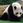Quantum Algorithm Zooの近似・シミュレーションアルゴリズムを見る に参加を申し込みました！Quantum Algorithm Zooの近似・シミュレーションアルゴリズムを見るに参加を申し込みました！Quantum Algorithm Zooの近似・シミュレーションアルゴリズムを見るに参加を申し込みました！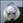Quantum Algorithm Zooの近似・シミュレーションアルゴリズムを見るに参加を申し込みました！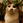Quantum Algorithm Zooの近似・シミュレーションアルゴリズムを見るに参加を申し込みました！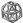Quantum Algorithm Zooの近似・シミュレーションアルゴリズムを見るに参加を申し込みました！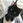Quantum Algorithm Zooの近似・シミュレーションアルゴリズムを見る に参加を申し込みました！Quantum Algorithm Zooの近似・シミュレーションアルゴリズムを見るに参加を申し込みました！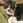Quantum Algorithm Zooの近似・シミュレーションアルゴリズムを見る に参加を申し込みました！